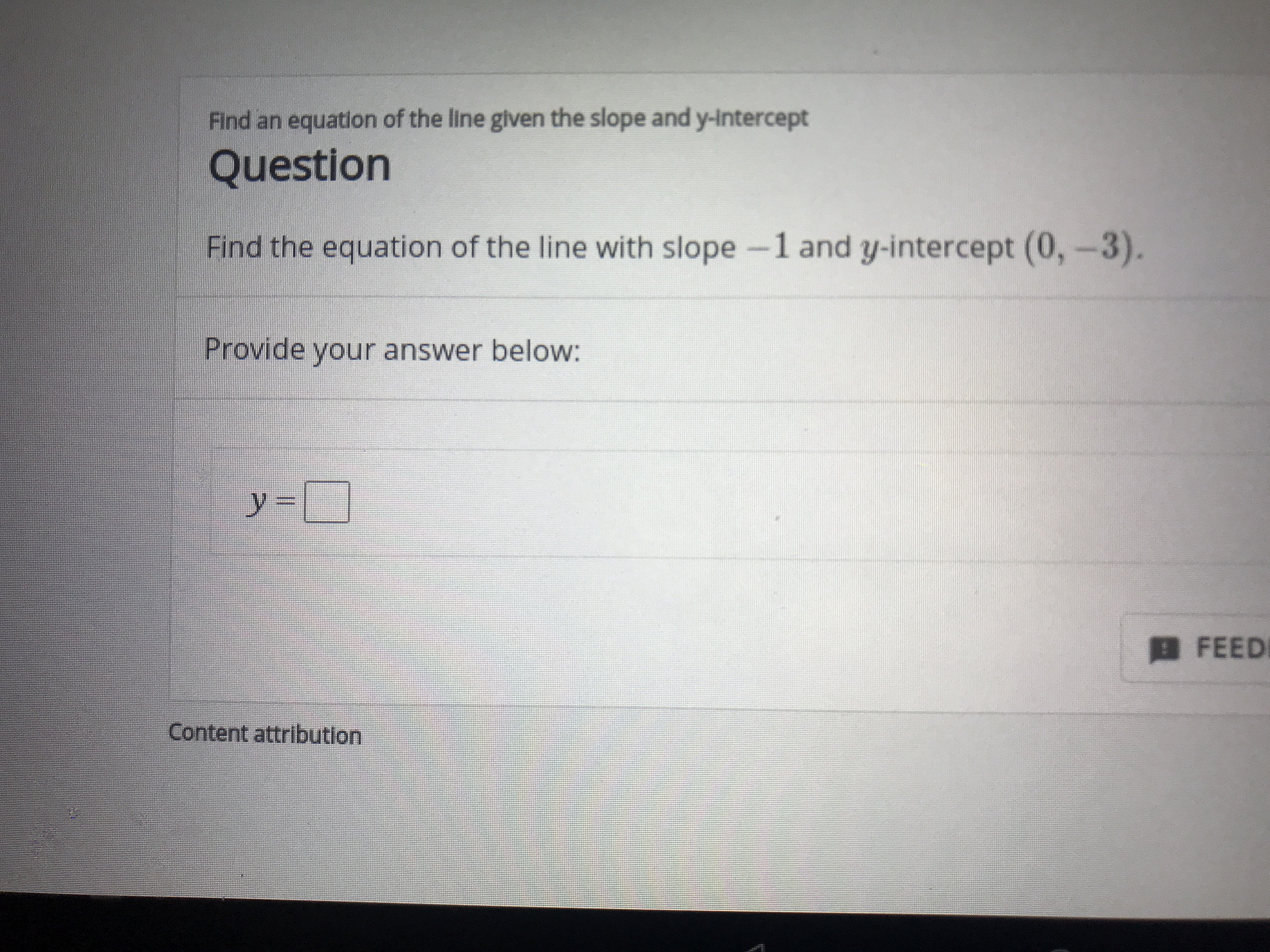# Find an equation of the line given the slope and y-InterceptQuestionFind the equation of the line with slope -1 and y-intercept (0,-3).Provide your answer belowy =FEEDContent attribution

Question
17 viewshelp_outlineImage TranscriptioncloseFind an equation of the line given the slope and y-Intercept Question Find the equation of the line with slope -1 and y-intercept (0,-3). Provide your answer below y = FEED Content attribution fullscreen
check_circle

Step 1

We will use slope intercept form. y=mx+b

m=...

### Want to see the full answer?

See Solution

#### Want to see this answer and more?

Solutions are written by subject experts who are available 24/7. Questions are typically answered within 1 hour.*

See Solution
*Response times may vary by subject and question.
Tagged in

### Other